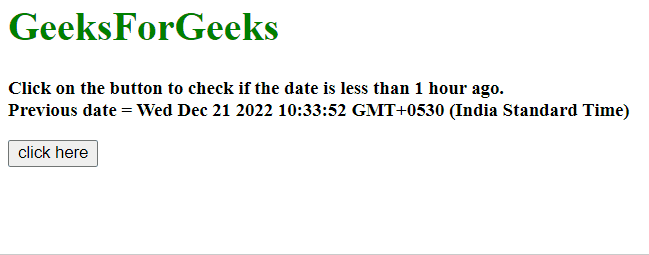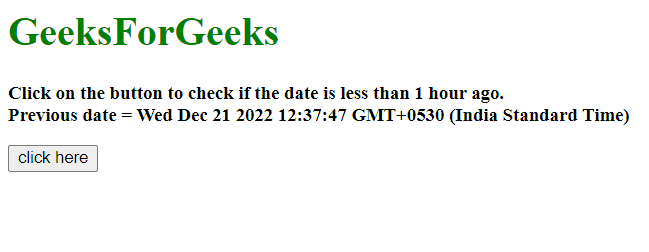# How to check if date is less than 1 hour ago using JavaScript ?

Given a date and the task is to check whether the given date is less than 1 hour ago or not with the help of JavaScript.

Approach 1:

• Count the milliseconds of the difference between the current and prev_date.
• If those are greater than milliseconds in 1 hour, then it returns false otherwise returns true.

Example: This example implements the above approach.

## html

 `<``h1` `style``=``"color:green;"``> ` `    ``GeeksForGeeks ` ` ` ` `  `<``p` `id``=``"GFG_UP"` `style``=``"font-size: 15px; font-weight: bold;"``> ` ` ` ` `  `<``button` `onclick``=``"gfg_Run()"``> ` `    ``click here ` ` ` ` `  `<``p` `id``=``"GFG_DOWN"` `style="color:green; ` `        ``font-size: 20px; font-weight: bold;"> ` ` ` ` `  `<``script``> ` `    ``var el_up = document.getElementById("GFG_UP"); ` `    ``var el_down = document.getElementById("GFG_DOWN"); ` `    ``var prev_date = new Date(); ` `    ``var d = new Date(); ` `         `  `    ``Date.prototype.addHours = function(h) { ` `        ``this.setTime(this.getTime() + (h*60*60*1000)); ` `        ``return this; ` `    ``} ` `     `  `    ``prev_date.addHours(-2); ` `    ``el_up.innerHTML = "Click on the button to " ` `            ``+ "check if the date is less than " ` `            ``+ "1 hour ago.<``br``>Previous date = " ` `            ``+ prev_date; ` `     `  `    ``function gfg_Run() { ` `         `  `        ``// Hour in milliseconds ` `        ``var ONE_HOUR = 60 * 60 * 1000; ` `         `  `        ``if ((d - prev_date) < ``ONE_HOUR``) { ` `            ``el_down.innerHTML` `= ``"Date is less"` `                        ``+ " than 1 hour ago."; ` `        ``} ` `        ``else { ` `            ``el_down.innerHTML` `= ``"Date is not "` `                    ``+ "less than 1 hour ago."; ` `        ``} ` `    ``}         ` ``

Output:Approach 2:

• Subtract 1 hour milliseconds from current time.
• Compare the milliseconds of the current and prev_date.
• If those are greater than milliseconds in 1 hour, then it return false otherwise returns true.

Example 2: This example uses the approach as discussed above.

## html

 `<``h1` `style``=``"color:green;"``> ` `    ``GeeksForGeeks ` ` ` ` `  `<``p` `id``=``"GFG_UP"` `style``=``"font-size: 15px; font-weight: bold;"``> ` ` ` ` `  `<``button` `onclick``=``"gfg_Run()"``> ` `    ``click here ` ` ` ` `  `<``p` `id``=``"GFG_DOWN"` `style="color:green; ` `        ``font-size: 20px; font-weight: bold;"> ` ` ` ` `  `<``script``> ` `    ``var el_up = document.getElementById("GFG_UP"); ` `    ``var el_down = document.getElementById("GFG_DOWN"); ` `    ``var prev_date = new Date(); ` `    ``var d = new Date(); ` `     `  `    ``const check = (date) => { ` `        ``const HOUR = 1000 * 60 * 60; ` `        ``const anHourAgo = Date.now() - HOUR; ` `        ``return date > anHourAgo; ` `    ``} ` `    ``el_up.innerHTML = "Click on the button to check" ` `                ``+ " if the date is less than 1 hour" ` `                ``+ " ago.<``br``>Previous date = " + prev_date; ` `     `  `    ``function gfg_Run() { ` `        ``var c = check(prev_date); ` `         `  `        ``if (c) { ` `            ``el_down.innerHTML = "Date is less " ` `                        ``+ "than 1 hour ago."; ` `        ``} ` `        ``else { ` `            ``el_down.innerHTML = "Date is not less" ` `                        ``+ " than 1 hour ago."; ` `        ``} ` `    ``}         ` ``

Output:Whether you're preparing for your first job interview or aiming to upskill in this ever-evolving tech landscape, GeeksforGeeks Courses are your key to success. We provide top-quality content at affordable prices, all geared towards accelerating your growth in a time-bound manner. Join the millions we've already empowered, and we're here to do the same for you. Don't miss out - check it out now!

Previous
Next